Hostname: page-component-5db6c4db9b-wnbrb Total loading time: 0 Render date: 2023-03-25T08:50:12.948Z Has data issue: true Feature Flags: { "useRatesEcommerce": false } hasContentIssue true

# ASYMPTOTIC ANALYSIS OF PERES’ ALGORITHM FOR RANDOM NUMBER GENERATION

Published online by Cambridge University Press:  12 October 2020

## Abstract

von Neumann [(1951). Various techniques used in connection with random digits. National Bureau of Standards Applied Math Series 12: 36–38] introduced a simple algorithm for generating independent unbiased random bits by tossing a (possibly) biased coin with unknown bias. While his algorithm fails to attain the entropy bound, Peres [(1992). Iterating von Neumann's procedure for extracting random bits. The Annals of Statistics 20(1): 590–597] showed that the entropy bound can be attained asymptotically by iterating von Neumann's algorithm. Let $b(n,p)$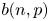denote the expected number of unbiased bits generated when Peres’ algorithm is applied to an input sequence consisting of the outcomes of $n$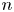tosses of the coin with bias $p$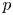. With $p=1/2$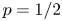, the coin is unbiased and the input sequence consists of $n$unbiased bits, so that $n-b(n,1/2)$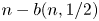may be referred to as the cost incurred by Peres’ algorithm when not knowing $p=1/2$. We show that $\lim _{n\to \infty }\log [n-b(n,1/2)]/\log n =\theta =\log [(1+\sqrt {5})/2]$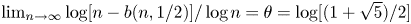(where $\log$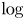is the logarithm to base $2$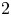), which together with limited numerical results suggests that $n-b(n,1/2)$may be a regularly varying sequence of index $\theta$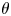. (A positive sequence $\{L(n)\}$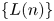is said to be regularly varying of index $\theta$if $\lim _{n\to \infty }L(\lfloor \lambda n\rfloor )/L(n)=\lambda ^\theta$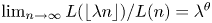for all $\lambda > 0$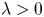, where $\lfloor x\rfloor$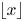denotes the largest integer not exceeding $x$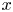.) Some open problems on the asymptotic behavior of $nh(p)-b(n,p)$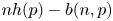are briefly discussed where $h(p)=-p\log p- (1-p)\log (1-p)$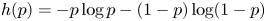denotes the Shannon entropy of a random bit with bias $p$.

Type
Research Article
Information

## Access options

Get access to the full version of this content by using one of the access options below. (Log in options will check for institutional or personal access. Content may require purchase if you do not have access.)

## References

Bojanic, R. & Seneta, E. (1973). A unified theory of regularly varying sequences. Mathematische Zeitschrift 134(2): 91106.CrossRefGoogle Scholar
Elias, P. (1972). The efficient construction of an unbiased random sequence. Annals of Mathematical Statistics 43(3): 865870.CrossRefGoogle Scholar
Jacquet, P. & Szpankowski, W. (1999). Entropy computations via analytic depoissonization. IEEE Transactions on Information Theory 45(4): 10721081.CrossRefGoogle Scholar
Kallenberg, O. (2002). Foundations of modern probability. New York: Springer.CrossRefGoogle Scholar
Keane, M.S. & O'Brien, G.L. (1994). A Bernoulli factory. ACM Transactions on Modeling and Computer Simulation 4(2): 213219.CrossRefGoogle Scholar
Nacu, Ş. & Peres, Y. (2005). Fast simulation of new coins from old. Annals of Applied Probability 15(1A): 93115.CrossRefGoogle Scholar
Pae, S.-i. (2013). Exact output rate of Peres's algorithm for random number generation. Information Processing Letters 113(5): 160164.CrossRefGoogle Scholar
Pae, S.-i. & Loui, M.C. (2006). Randomizing functions: simulation of a discrete probability distribution using a source of unknown distribution. IEEE Transactions on Information Theory 52(11): 49654976.CrossRefGoogle Scholar
Peres, Y. (1992). Iterating von Neumann's procedure for extracting random bits. The Annals of Statistics 20(1): 590597.CrossRefGoogle Scholar
Prasitsupparote, A., Konno, N., & Shikata, J. (2018). Numerical and non-asymptotic analysis of Elias's and Peres's extractors with finite input sequences. Entropy 20(10): Article ID 729, 19 pages.CrossRefGoogle ScholarPubMed
Ryabko, B. (1998). Fast enumeration of combinatorial objects. Discrete Mathematics and Applications 8(2): 163182.CrossRefGoogle Scholar
Ryabko, B.Y. & Matchikina, E. (2000). Fast and efficient construction of an unbiased random sequence. IEEE Transactions on Information Theory 46(3): 10901093.CrossRefGoogle Scholar
von Neumann, J. (1951). Various techniques used in connection with random digits. National Bureau of Standards Applied Math Series 12: 3638.Google Scholar
Wei, W. & Guo, H. (2009). Bias-free true random-number generator. Optics Letters 34(12): 18761878.CrossRefGoogle ScholarPubMed